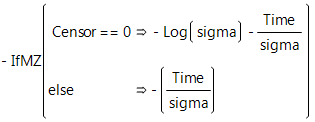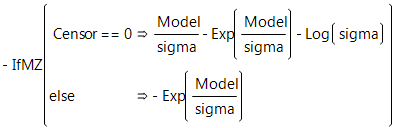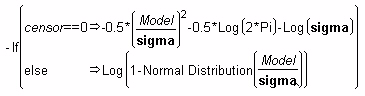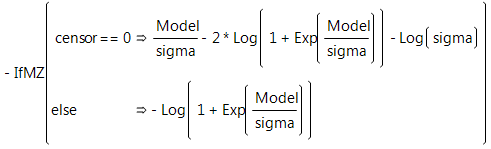Reliability and Survival Methods > Fit Parametric Survival > Statistical Details for the Fit Parametric Survival Platform
Publication date: 08/13/2020

# Statistical Details for the Fit Parametric Survival Platform

This section contains statistical details for the Fit Parametric Survival platform.

## Loss Formulas for Survival Distributions

The following formulas are for the negative log-likelihoods to fit common parametric models. Each formula uses the calculator if conditional function with the uncensored case of the conditional first and the right-censored case as the Else clause. You can copy these formulas from tables in the Loss Function Templates folder in Sample Data and paste them into your data table.

### Exponential Loss Function

In the exponential loss function shown here, sigma represents the mean of the exponential distribution and Time is the age at failure.

#### Exponential Loss FunctionA characteristic of the exponential distribution is that the instantaneous failure rate remains constant over time. This means that the chance of failure for any subject during a given length of time is the same regardless of how long a subject has been in the study.

### Weibull Loss Function

The Weibull density function often provides a good model for the lifetime distributions. You can use the Survival platform for an initial investigation of data to determine whether the Weibull loss function is appropriate for your data.

#### Weibull Loss FunctionThere are examples of one-parameter, two-parameter, and extreme-value functions in the Loss Function Templates folder.

### Lognormal Loss Function

The formula shown below is the lognormal loss function where Normal Distribution(model/sigma) is the standard normal distribution function. The hazard function has value 0 at t = 0, increases to a maximum, and then decreases. The hazard function approaches zero as t becomes large.

#### Lognormal Loss Function### Loglogistic Loss Function

If Y is distributed as the logistic distribution, Exp(Y) is distributed as the loglogistic distribution.

#### Loglogistic Loss Function